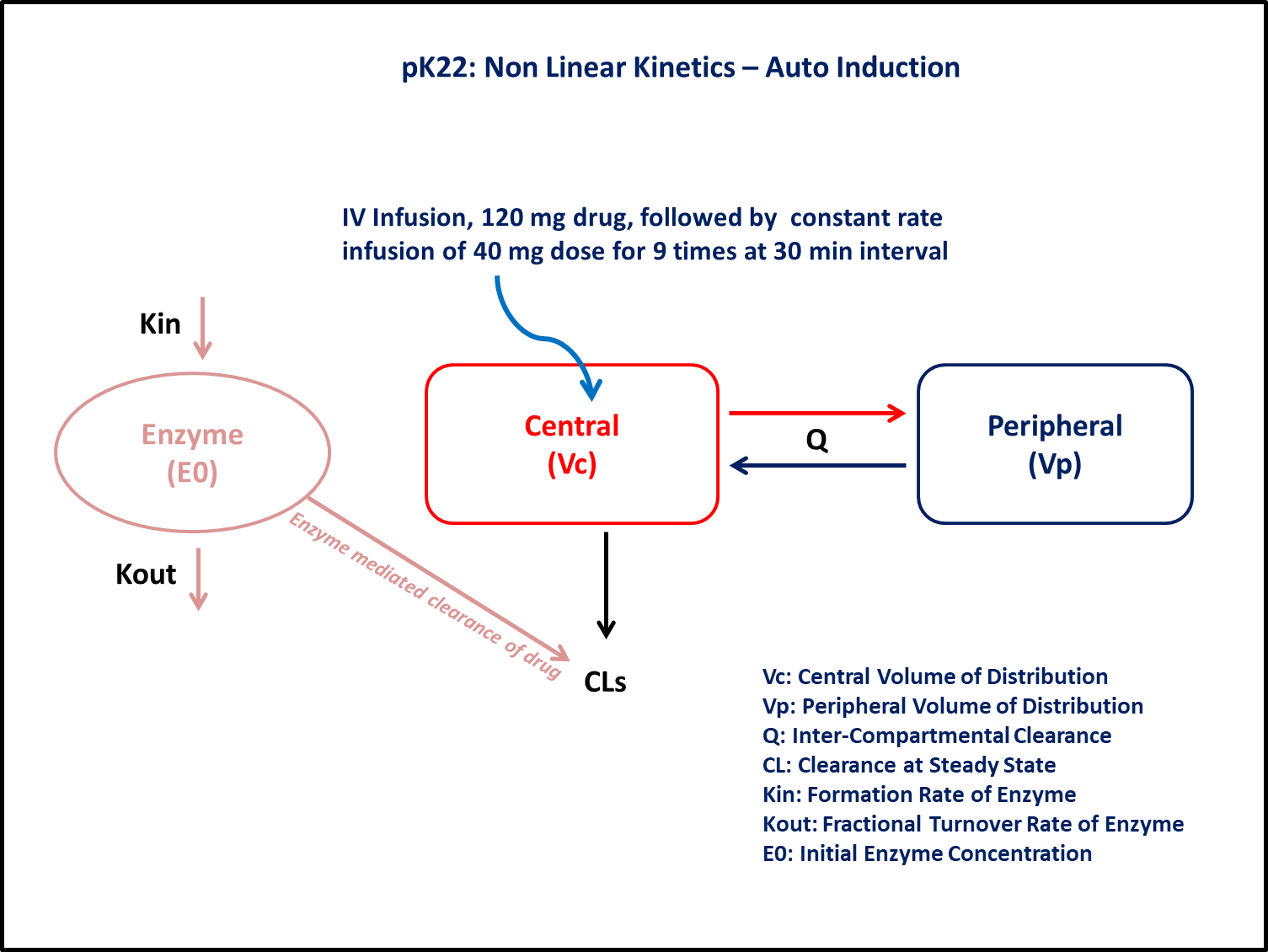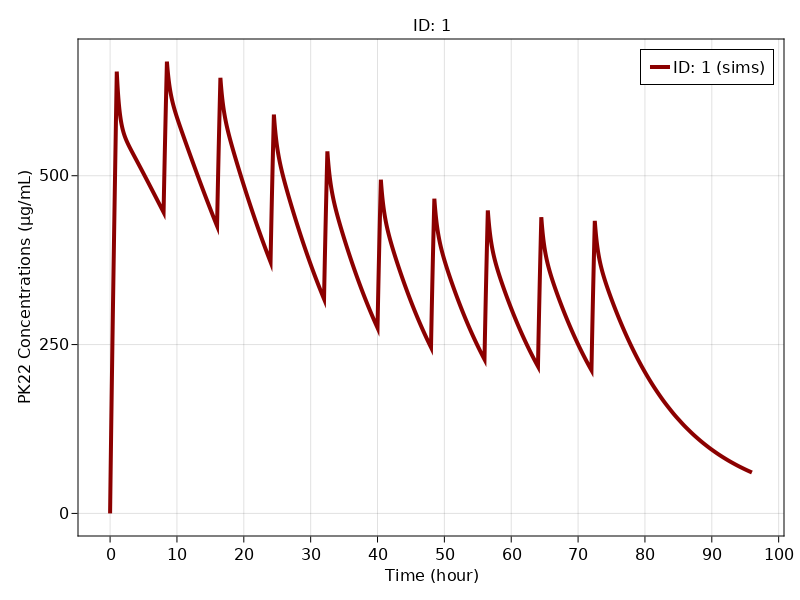# Exercise PK22 - Non Linear Kinetics - Auto Induction

### Background

• Structural model - Two compartment Model for drug and one compartment model for enzyme

• Route of administration - IV Infusion

• Dosage Regimen - 120 mg of IV infusion with constant rate of 1 hr followed by 9 more doses of 40 mg for 30 minutes duration at the interval of 8 hrs

• Number of Subjects - 1### Learning Outcome

This model gives an understanding of drug pharmacokinetics and enzyme autoinduction simultaneously after repeated IV infusion.

### Objectives

In this tutorial, you will learn how to build a two compartment model for a drug and one compartment model for an enzyme and simulate from the model.

### Libraries

Call the "necessary" libraries to get started.

using Random
using Pumas
using PumasUtilities
using CairoMakie


### Model

In this two compartment model, we administer repeated dose of IV infusion to a single subject.

pk_22            = @model  begin
desc = "Autoinduction Model"
timeu = u"hr"
end

@param begin
tvcls       ∈ RealDomain(lower=0)
"Central Volume of Distribution (L)"
tvvc        ∈ RealDomain(lower=0)
"Peripheral Volume of Distribution (L)"
tvvp        ∈ RealDomain(lower=0)
"Distribution Clearance (L/hr)"
tvq         ∈ RealDomain(lower=0)
"Input Rate Constant for Enzyme (hr⁻¹)"
tvkin       ∈ RealDomain(lower=0)
"Output Rate Constant for Enzyme (hr⁻¹)"
tvkout      ∈ RealDomain(lower=0)
"Initial Enzyme Concentration"
tvE0        ∈ RealDomain(lower=0)
Ω           ∈ PDiagDomain(7)
"Proportional RUV"
σ²_prop     ∈ RealDomain(lower=0)
end

@random begin
η           ~ MvNormal(Ω)
end

@pre begin
Cls         = tvcls * exp(η)
Vc          = tvvc * exp(η)
Vp          = tvvp * exp(η)
Q           = tvq * exp(η)
Kin         = tvkin * exp(η)
Kout        = tvkout * exp(η)
E0          = tvE0 * exp(η)
end

@init begin
Enzyme      = (Kin/Kout)+ E0
end

@dynamics begin
Central'    = -(Cls/Vc) * Central * Enzyme + (Q/Vp) * Peripheral - (Q/Vc) * Central
Peripheral' =  (Q/Vc) * Central - (Q/Vp) * Peripheral
Enzyme'     =  Kin * (E0+Central/Vc) -  Kout * Enzyme
end

@derived begin
cp          = @. Central/Vc
"""
Observed Concentration (mcg/L))
"""
dv          ~ @. Normal(cp, sqrt(cp^2*σ²_prop))
end
end

PumasModel
Parameters: tvcls, tvvc, tvvp, tvq, tvkin, tvkout, tvE0, Ω, σ²_prop
Random effects: η
Covariates:
Dynamical variables: Central, Peripheral, Enzyme
Derived: cp, dv
Observed: cp, dv


### Parameters

Hereby Parameters are provided for the simulation. tv represents the typical value for parameters.

• $tvcls$ - Typical Value Clearance at Steady State (L/hr)

• $tvvc$ - Typical Value Central Volume of Distribution (L)

• $tvvp$ - Typical Value Peripheral Volume of Distribution (L)

• $tvq$ - Typical Value Distribution Clearance (L/hr)

• $tvkin$ - Input Rate Constant for Enzyme (hr⁻¹)

• $tvkout$ - Output Rate Constant for Enzyme (hr⁻¹)

• $tvE0$ - Initial Enzyme Concentration

• $Ω$ - Between Subject Variability

• $σ$ - Residual errors

param =  (tvcls    = 0.04,
tvvc     = 150.453,
tvvp     = 54.0607,
tvq      = 97.8034,
tvkin    = 0.0238896,
tvkout   = 0.0238896,
tvE0     = 132.864,
Ω        = Diagonal([0.0,0.0,0.0,0.0,0.0,0.0,0.0]),
σ²_prop  = 0.005)

(tvcls = 0.04, tvvc = 150.453, tvvp = 54.0607, tvq = 97.8034, tvkin = 0.023
8896, tvkout = 0.0238896, tvE0 = 132.864, Ω = [0.0 0.0 … 0.0 0.0; 0.0 0.0 …
0.0 0.0; … ; 0.0 0.0 … 0.0 0.0; 0.0 0.0 … 0.0 0.0], σ²_prop = 0.005)


### Dosage Regimen

Dosage Regimen - 120 mg of IV infusion with constant rate of 1 hr followed by 9 more doses of 40 mg for 30 minutes duration at the interval of 8 hrs

ev1  = DosageRegimen([120000,40000], time = [0,8], duration = [1,0.5],
cmt = [1,1], addl = [0,8], ii = [0,8])
sub1 = Subject(id = 1, events = ev1, observations = (cp = nothing,))

Subject
ID: 1
Events: 20
Observations: cp: (nothing)


### Simulation

Lets simulate for plasma concentration with the specific observation time points after IV.

Random.seed!(123)
sim_sub1 = simobs(pk_22, sub1, param, obstimes=0.00:0.01:96)


### Visualization

f, a, p = sim_plot(pk_22, [sim_sub1],
observations = :cp,
color = :redsblues,
linewidth = 4,
axis = (xlabel = "Time (hour)",
ylabel = "PK22 Concentrations (μg/mL)",
xticks = 0:10:100))
axislegend(a)
f